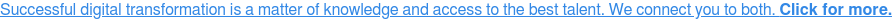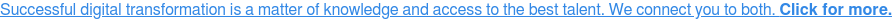# Beyond Modeling 101

Anyone working in marketing research or data science has had some exposure to statistical modeling. This might be quite basic – linear regression and ANOVA in an introductory statistics class for example – or extensive – a PhD in mathematical statistics for instance.

For those in the first group, the following is a snapshot of what comes next. It has been adapted from the Mplus statistical software documentation. The models listed are popular in many fields but by no means is this list all-encompassing.At one point or another, I have used most of them. If you’d like to learn more about any of these methods, there is a wealth of information on the Web about statistical topics. UC Berkeley’s Glossary of Statistical Terms is one authoritative source.

There are three important distinctions that need to be made about the variables in an analysis in order to be able to specify a model. The distinctions are whether variables are observed or latent, whether variables are dependent or independent, and the scale of the observed dependent variables.

Two types of variables can be modelled: observed variables and latent variables. Observed variables are variables that are directly-measured such as test scores and diagnostic criteria. They are sometimes referred to as manifest variables, outcomes, or indicators. Latent variables are variables that are not directly measured such as ability, depression, and health status. They are measured indirectly by a set of observed variables.There are two types of latent variables: continuous and categorical. Continuous latent variables are sometimes referred to as factors, dimension, constructs, or random effects. Categorical latent variables are sometimes referred to as latent class variables or mixtures.

Observed and latent variables can play the role of a dependent variable or an independent variable in the model. The distinction between dependent and independent variables is that of a regression analysis for y regressed on x where y is a dependent variable and x is an independent variable. An independent variable is one that is not influenced by any other variable.

Dependent variables are those that are influenced by other variables. Other terms used for dependent variables are outcome variable, response variable, indicator variable, y variable, and endogenous variable. Other terms used for independent variables are covariate, background variable, explanatory variable, predictor, x variable, and exogenous variable.The scale of observed dependent variables can be continuous, censored, binary, ordered categorical (ordinal), unordered categorical (nominal), counts, or combinations of these variable types.

Listed below are some general kinds of statistical models:

• Categorical regression analysis
• Path analysis
• Exploratory factor analysis
• Confirmatory factor analysis
• Item response theory modeling
• Structural equation modeling
• Growth modeling
• Discrete-time survival analysis
• Continuous-time survival analysis
• Time series analysis

Some variations of these models include:

• Factor mixture modeling
• Structural equation mixture modeling
• Loglinear modeling
• Regression mixture modeling
• Path analysis mixture modeling
• Growth mixture modeling with latent trajectory classes
• Latent class analysis with covariates and direct effects
• Latent class analysis with multiple categorical latent variables
• Complier Average Causal Effect (CACE) modeling
• Discrete-time survival mixture analysis
• Continuous-time survival mixture analysis
• Multiple group analysis

Adapted from Version 8 Mplus User’s GuideAny copy/paste and editing errors are mine.

Browse

Article by channel:

Read more articles tagged: Featured, Marketing Analytics